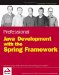# List of Figures

Figure 1-1

Figure 2-1

Figure 4-1
Figure 4-2
Figure 4-3

Figure 5-1
Figure 5-2
Figure 5-3
Figure 5-4
Figure 5-5

Figure 6-1
Figure 6-2

Figure 10-1
Figure 10-2
Figure 10-3
Figure 10-4
Figure 10-5
Figure 10-6
Figure 10-7
Figure 10-8

Figure 12-1
Figure 12-2
Figure 12-3
Figure 12-4
Figure 12-5
Figure 12-6
Figure 12-7
Figure 12-8

Figure 13-1
Figure 13-2

Figure 14-1

Figure 15-1
Figure 15-2
Figure 15-3
Figure 15-4
Figure 15-5
Figure 15-6
Figure 15-7
Figure 15-8

## Appendix A: Requirements for the Sample Application

Figure A-1
Figure A-2
Figure A-3
Figure A-4
Figure A-5
Figure A-6
Figure A-7
Figure A-8Professional Java Development with the Spring Framework
ISBN: 0764574833
EAN: 2147483647
Year: 2003
Pages: 188